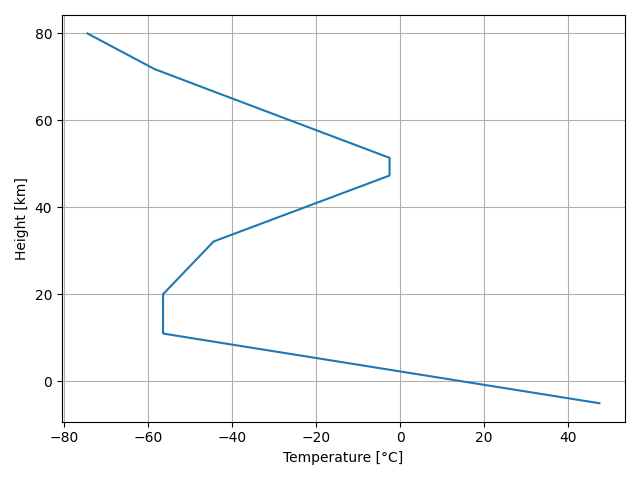A full implementation of the ICAO standard atmosphere 1993

## Project descriptionAmbiance is a full implementation of the ICAO standard atmosphere 1993 written in Python.

## Basic usage

Atmospheric properties are computed from an Atmosphere object which takes the altitude (geometric height) as input. For instance, to simply retrieve sea level properties, you can write:

>>> from ambiance import Atmosphere
>>> sealevel = Atmosphere(0)

>>> sealevel.temperature
array([288.15])

>>> sealevel.pressure
array([101325.])

>>> sealevel.kinematic_viscosity
array([1.46071857e-05])

### List of available atmospheric properties

• Collision frequency (collision_frequency)

• Density (density)

• Dynamic viscosity (dynamic_viscosity)

• Geometric height above MSL (h)

• Geopotential height (H)

• Gravitational acceleration (grav_accel)

• Kinematic viscosity (kinematic_viscosity)

• Layer names (layer_name) [string array]

• Mean free path (mean_free_path)

• Mean particle speed (mean_particle_speed)

• Number density (number_density)

• Pressure (pressure)

• Pressure scale height (pressure_scale_height)

• Specific weight (specific_weight)

• Speed of sound (speed_of_sound)

• Temperature (temperature, temperature_in_celsius)

• Thermal conductivity (thermal_conductivity)

### Vector and matrix inputs

Ambiance also handles list-like input (list, tuples, Numpy arrays). The following code demonstrates how to produce a temperature plot with Matplotlib. In the example, Numpy’s linspace() function is used to produce an array with altitudes.

import numpy as np
import matplotlib.pyplot as plt
from ambiance import Atmosphere

# Create an atmosphere object
heights = np.linspace(-5e3, 80e3, num=1000)
atmosphere = Atmosphere(heights)

# Make plot
plt.plot(atmosphere.temperature_in_celsius, heights/1000)
plt.ylabel('Height [km]')
plt.xlabel('Temperature [°C]')
plt.grid()
plt.show()

The output isSimilarly, you can also pass in entire matrices. Example:

>>> import numpy as np
>>> from ambiance import Atmosphere

>>> h = np.array([[0, 11, 12], [20, 21, 35], [0, 80, 50]])*1000
>>> h  # Geometric heights in metres
array([[    0, 11000, 12000],
[20000, 21000, 35000],
[    0, 80000, 50000]])

>>> Atmosphere(h).temperature
array([[288.15      , 216.7735127 , 216.65      ],
[216.65      , 217.58085353, 236.51337209],
[288.15      , 198.63857625, 270.65      ]])

>>> Atmosphere(h).speed_of_sound
array([[340.29398803, 295.15359145, 295.06949351],
[295.06949351, 295.70270856, 308.29949587],
[340.29398803, 282.53793156, 329.798731  ]])

>>> Atmosphere([30000, 0]).layer_name
array(['stratosphere', 'troposphere'], dtype='<U42')

### Instantiating from given pressure or density

In some contexts it may be convenient to instantiate an Atmosphere object from a given ambient pressure or density. This can be easily achieved by using the Atmosphere.from_pressure() or Atmosphere.from_density() methods, respectively. Both methods return Atmosphere objects from which all other properties, like temperature, can be requested.

>>> Atmosphere.from_pressure([80e3, 20e3])  # 80 kPa and 20 kPa
Atmosphere(array([ 1949.58557497, 11805.91571135]))

>>> Atmosphere.from_pressure([80e3, 20e3]).pressure
array([80000., 20000.])

>>> Atmosphere.from_density(1.0)  # 1.0 kg/m^3
Atmosphere(array([2064.96635895]))

### Complete user guide

For a comprehensive and detailed user guide, please see the complete documentation.

## Installation

### Conda

The package can be installed via the Conda environment with

conda install -c conda-forge ambiance## Requirements

Using Ambiance requires

• Python 3.6 or higher

• NumPy

• SciPy

For developers: Recommended packages may be installed with the requirements.txt.

pip install -r requirements.txt

## Project details

This version1.3.11.3.01.2.01.1.21.1.01.0.21.0.11.0.00.3.20.3.10.3.00.2.10.2.00.1.0

Uploaded source
Uploaded py3